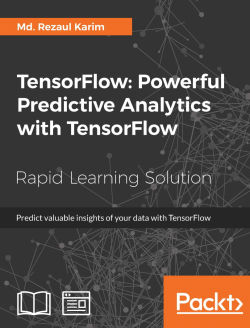# TensorFlow: Powerful Predictive Analytics with TensorFlow4.5 (2 reviews total)
By Md. Rezaul Karim
• FREE Subscription Access now
• \$12.99 eBook + Subscription
What do you get with a Packt Subscription?

• Constantly updated with 100+ new titles each month
• Breadth and depth in over 1,000+ technologies

Predictive analytics discovers hidden patterns from structured and unstructured data for automated decision making in business intelligence. Predictive decisions are becoming a huge trend worldwide, catering to wide industry sectors by predicting which decisions are more likely to give maximum results. TensorFlow, Google’s brainchild, is immensely popular and extensively used for predictive analysis.

This book is a quick learning guide on all the three types of machine learning, that is, supervised, unsupervised, and reinforcement learning with TensorFlow. This book will teach you predictive analytics for high-dimensional and sequence data. In particular, you will learn the linear regression model for regression analysis. You will also learn how to use regression for predicting continuous values. You will learn supervised learning algorithms for predictive analytics. You will explore unsupervised learning and clustering using K-meansYou will then learn how to predict neighborhoods using K-means, and then, see another example of clustering audio clips based on their audio features.

This book is ideal for developers, data analysts, machine learning practitioners, and deep learning enthusiasts who want to build powerful, robust, and accurate predictive models with the power of TensorFlow.

This book is embedded with useful assessments that will help you revise the concepts you have learned in this book.

Publication date:
March 2018
Publisher
Packt
Pages
164
ISBN
9781789136913

## Chapter 1. From Data to Decisions – Getting Started with TensorFlow

Despite the huge availability of data and significant investments, many business organizations still go on gut feel because they neither make the proper use of the data nor do they take appropriate and effective business decisions. TensorFlow, on the other hand, can be used to help take the business decision from this huge collection of data. TensorFlow is mathematical software and an open source software library for Machine Intelligence, developed in 2011 by the Google Brain Team and it can be used to help us analyze data to predict the effective business outcome. Although the initial target of TensorFlow was to conduct research in machine learning and in deep neural networks, however, the system is general enough to be applicable in a wide variety of other domains as well.

Keeping in mind your needs and based on all the latest and exciting features of TensorFlow 1.x, in this lesson, we will give a description of the main TensorFlow capabilities that are mostly motivated by a real-life example using the data.

The following topics will be covered in this lesson:

• From data to decision: Titanic example

• General overview of TensorFlow

• Installing and configuring TensorFlow

• TensorFlow computational graph

• TensorFlow programming model

• TensorFlow data model

• Visualizing through TensorBoard

• Getting started with TensorFlow: linear regression and beyond

## Taking Decisions Based on Data – Titanic Example

The growing demand for data is a key challenge. Decision support teams such as institutional research and business intelligence often cannot take the right decisions on how to expand their business and research outcomes from a huge collection of data. Although data plays an important role in driving the decision, however, in reality, taking the right decision at right time is the goal.

In other words, the goal is the decision support, not the data support. This can be achieved through an advanced use of data management and analytics.

### Data Value Chain for Making Decisions

The following diagram in figure 1 (source: H. Gilbert Miller and Peter Mork, From Data to Decisions: A Value Chain for Big Data, Proc. Of IT Professional, Volume: 15, Issue: 1, Jan.-Feb. 2013, DOI: 10.1109/MITP.2013.11) shows the data chain towards taking actual decisions–that is, the goal. The value chains start through the data discovery stage consisting of several steps such as data collection and annotating data preparation, and then organizing them in a logical order having the desired flow. Then comes the data integration for establishing a common data representation of the data. Since the target is to take the right decision, for future reference having the appropriate provenance of the data–that is, where it comes from, is important: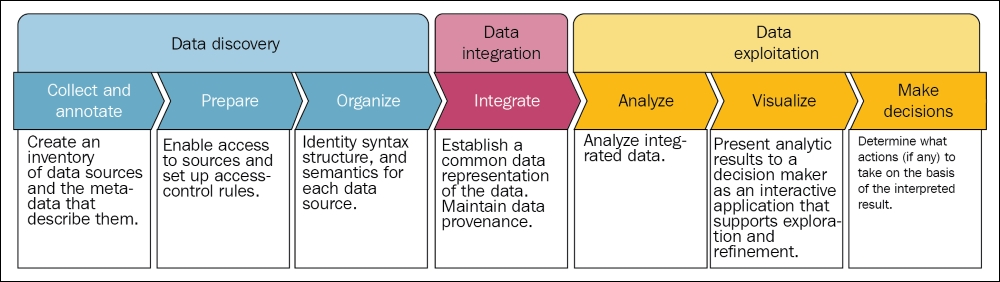Figure 1: From data to decisions: a value chain for big data

Well, now your data is somehow integrated into a presentable format, it's time for the data exploration stage, which consists of several steps such as analyzing the integrated data and visualization before taking the actions to take on the basis of the interpreted results.

However, is this enough before taking the right decision? Probably not! The reason is that it lacks enough analytics, which eventually helps to take the decision with an actionable insight. Predictive analytics comes in here to fill the gap between. Now let's see an example of how in the following section.

### From Disaster to Decision – Titanic Survival Example

Here is the challenge, Titanic–Machine Learning from Disaster from Kaggle (https://www.kaggle.com/c/titanic):

"The sinking of the RMS Titanic is one of the most infamous shipwrecks in history. On April 15, 1912, during her maiden voyage, the Titanic sank after colliding with an iceberg, killing 1502 out of 2224 passengers and crew. This sensational tragedy shocked the international community and led to better safety regulations for ships. One of the reasons that the shipwreck led to such loss of life was that there were not enough lifeboats for the passengers and crew. Although there was some element of luck involved in surviving the sinking, some groups of people were more likely to survive than others, such as women, children, and the upper-class. In this challenge, we ask you to complete the analysis of what sorts of people were likely to survive. In particular, we ask you to apply the tools of machine learning to predict which passengers survived the tragedy."

But going into this deeper, we need to know about the data of passengers travelling in the Titanic during the disaster so that we can develop a predictive model that can be used for survival analysis.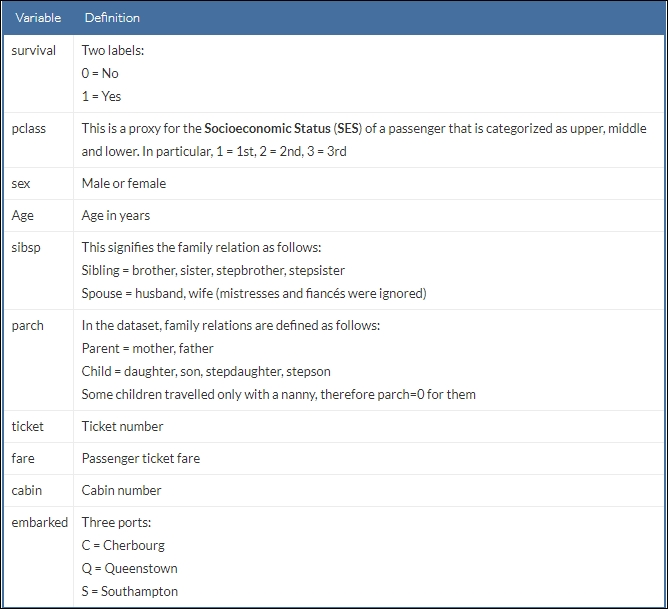A snapshot of the dataset can be seen as follows: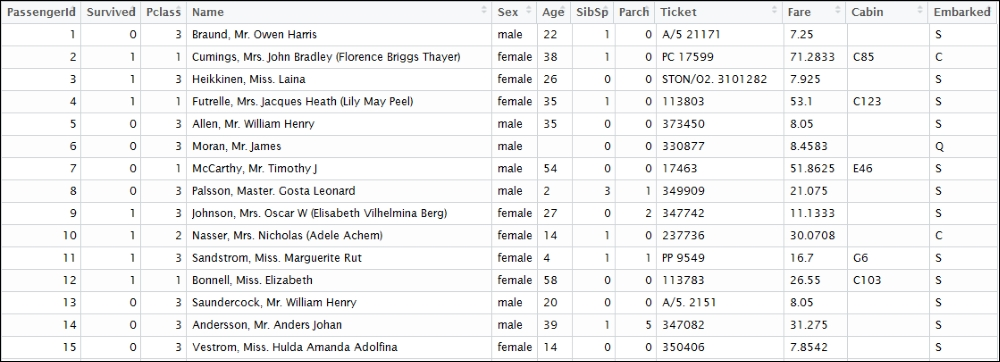Figure 2: A snapshot of the Titanic survival dataset

The ultimate target of using this dataset is to predict what kind of people survived the Titanic disaster. However, a bit of exploratory analysis of the dataset is a mandate. At first, we need to import necessary packages and libraries:

```import pandas as pd
import matplotlib.pyplot as plt
import numpy as np```

Now read the dataset and create a panda's DataFrame:

`df = pd.read_csv('/home/asif/titanic_data.csv')`

Before drawing the distribution of the dataset, let's specify the parameters for the graph:

```fig = plt.figure(figsize=(18,6), dpi=1600)
alpha=alpha_scatterplot = 0.2
alpha_bar_chart = 0.55
fig = plt.figure()

Draw a bar diagram for showing who survived versus who did not:

```ax1 = plt.subplot2grid((2,3),(0,0))
ax1.set_xlim(-1, 2)
df.Survived.value_counts().plot(kind='bar', alpha=alpha_bar_chart)
plt.title("Survival distribution: 1 = survived")```

Plot a graph showing survival by `Age`:

```plt.subplot2grid((2,3),(0,1))
plt.scatter(df.Survived, df.Age, alpha=alpha_scatterplot)
plt.ylabel("Age")
plt.grid(b=True, which='major', axis='y')
plt.title("Survival by Age: 1 = survived")```

Plot a graph showing distribution of the `passengers` classes:

```ax3 = plt.subplot2grid((2,3),(0,2))
df.Pclass.value_counts().plot(kind="barh", alpha=alpha_bar_chart)
ax3.set_ylim(-1, len(df.Pclass.value_counts()))
plt.title("Class dist. of the passengers")```

Plot a kernel density estimate of the subset of the 1st class passengers' age:

```plt.subplot2grid((2,3),(1,0), colspan=2)
df.Age[df.Pclass == 1].plot(kind='kde')
df.Age[df.Pclass == 2].plot(kind='kde')
df.Age[df.Pclass == 3].plot(kind='kde')
plt.xlabel("Age")
plt.title("Age dist. within class")
plt.legend(('1st Class', '2nd Class','3rd Class'),loc='best')```

Plot a graph showing `passengers per boarding location`:

```ax5 = plt.subplot2grid((2,3),(1,2))
df.Embarked.value_counts().plot(kind='bar', alpha=alpha_bar_chart)
ax5.set_xlim(-1, len(df.Embarked.value_counts()))
plt.title("Passengers per boarding location")
Finally, we show all the subplots together:
plt.show()
>>>```

The figure shows the survival distribution, survival by age, age distribution, and the passengers per boarding location: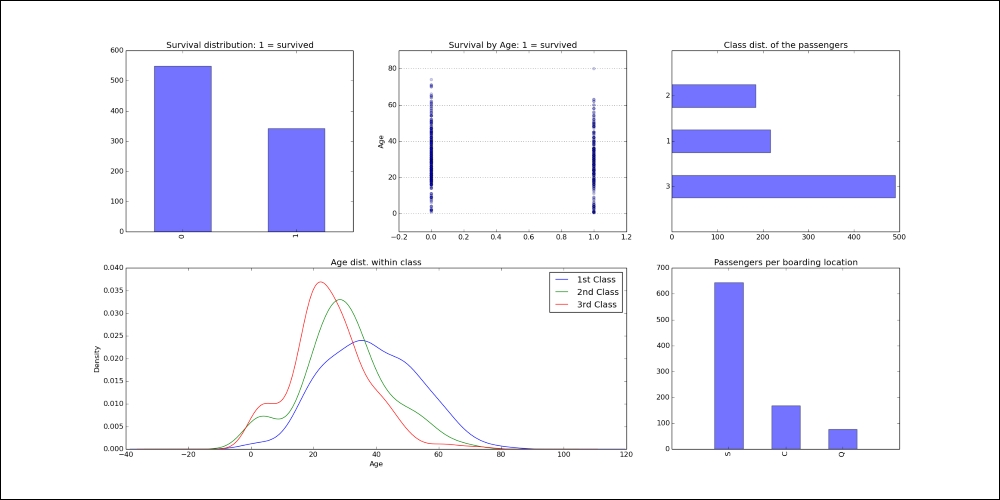Figure 3: Titanic survival data distribution across age, class, and age within classes and boarding location

However, to execute the preceding code, you need to install several packages such as matplotlib, pandas, and scipy. They are listed as follows:

• Installing pandas: Pandas is a Python package for data manipulation. It can be installed as follows:

```\$ sudo pip3 install pandas
#For Python 2.7, use the following:
\$ sudo pip install pandas
```
• Installing matplotlib: In the preceding code, matplotlib is a plotting library for mathematical objects. It can be installed as follows:

```\$ sudo apt-get install python-matplotlib   # for Python 2.7
\$ sudo apt-get install python3-matplotlib # for Python 3.x
```
• Installing scipy: Scipy is a Python package for scientific computing. Installing `blas` and `lapack`and `gfortran` are a prerequisite for this one. Now just execute the following command on your terminal:

```\$ sudo apt-get install libblas-dev liblapack-dev \$ sudo apt-get install gfortran \$ sudo pip3 install scipy # for Python 3.x
\$ sudo pip install scipy # for Python 2.7
```

For Mac, use the following command to install the above modules:

```\$ sudo easy_install pip
\$ sudo pip install matplotlib
\$ sudo pip install libblas-dev liblapack-dev
\$ sudo pip install gfortran
\$ sudo pip install scipy
```

For windows, I am assuming that Python 2.7 is already installed at C:\Python27. Then open the command prompt and type the following command:

```C:\Users\admin-karim>cd C:/Python27
C:\Python27> python -m pip install <package_name> # provide package name accordingly.
```

For Python3, issue the following commands:

```C:\Users\admin-karim>cd C:\Users\admin-karim\AppData\Local\Programs\Python\Python35\Scripts
```

Well, we have seen the data. Now it's your turn to do some analytics on top of the data. Say predicting what kinds of people survived from that disaster. Don't you agree that we have enough information about the passengers, but how could we do the predictive modeling so that we can draw some fairly straightforward conclusions from this data?

For example, say being a woman, being in 1st class, and being a child were all factors that could boost passenger chances of survival during this disaster.

In a brute-force approach–for example, using if/else statements with some sort of weighted scoring system, you could write a program to predict whether a given passenger would survive the disaster. However, does writing such a program in Python make much sense? Naturally, it would be very tedious to write, difficult to generalize, and would require extensive fine tuning for each variable and samples (that is, passenger).

This is where predictive analytics with machine learning algorithms and emerging tools comes in so that you could build a program that learns from the sample data to predict whether a given passenger would survive. In such cases, we will see throughout this book that TensorFlow could be a perfect solution to achieve outstanding accuracies across your predictive models. We will start describing the general overview of the TensorFlow framework. Then we will show how to install and configure TensorFlow on Linux, Mac OS and Windows.

## General Overview of TensorFlow

TensorFlow is an open source framework from Google for scientific and numerical computation based on dataflow graphs that stand for the TensorFlow's execution model. The dataflow graphs used in TensorFlow help the machine learning experts to perform more advanced and intensive training on the data for developing deep learning and predictive analytics models. In 2015, Google open sourced the TensorFlow and all of its reference implementation and made all the source code available on GitHub under the Apache 2.0 license. Since then, TensorFlow has achieved wide adoption from academia and research to the industry, and following that recently the most stable version 1.x has been released with a unified API.

As the name TensorFlow implies, operations are performed by neural networks on multidimensional data arrays (aka flow of tensors). This way, TensorFlow provides some widely used and robust implementation linear models and deep learning algorithms.

Deploying a predictive or general purpose model using TensorFlow is pretty straightforward. The thing is that once you have constructed your neural networks model after necessary feature engineering, you can simply perform the training interactively using plotting or TensorBoard (we will see more on it in upcoming sections). Finally, you deploy it eventually after evaluating it by feeding it some test data.

Since we are talking about the dataflow graphs, nodes in a flow graph correspond to the mathematical operations, such as addition, multiplication, matrix factorization, and so on, whereas, edges correspond to tensors that ensure communication between edges and nodes, that is dataflow and controlflow.

You can perform the numerical computation on a CPU. Nevertheless, using TensorFlow, it is also possible to distribute the training across multiple devices on the same system and train on them, especially if you have more than one GPU on your system so that these can share the computational load. But the precondition is if TensorFlow can access these devices, it will automatically distribute the computations to the multiple devices via a greedy process. But TensorFlow also allows the program, to specify which operations will be on which devices via name scope placement.

The APIs in TensorFlow 1.x have changed in ways that are not all backward compatible. That is, TensorFlow programs that worked on TensorFlow 0.x won't necessarily work on TensorFlow 1.x.

The main features offered by the latest release of TensorFlow are:

• Faster computing: The latest release of TensorFlow is incredibly faster. For example, it is 7.3 times faster on 8 GPUs for Inception v3 and 58 times speedup for distributed inception (v3 training on 64 GPUs).

• Flexibility: TensorFlow is not just a deep learning library, but it comes with almost everything you need for powerful mathematical operations through functions for solving the most difficult problems. TensorFlow 1.x introduces some high-level APIs for high-dimensional arrays or tensors, with `tf.layers`, `tf.metrics`, `tf.losses`, and `tf.keras` modules. These have made TensorFlow very suitable for high-level neural networks computing.

• Portability: TensorFlow runs on Windows, Linux, and Mac machines and on mobile computing platforms (that is, Android).

• Easy debugging: TensorFlow provides the TensorBoard tool for the analysis of the developed models.

• Unified API: TensorFlow offers you a very flexible architecture that enables you to deploy computation to one or more CPUs or GPUs in a desktop, server, or mobile device with a single API.

• Transparent use of GPU computing: Automating management and optimization of the same memory and the data used. You can now use your machine for large-scale and data-intensive GPU computing with NVIDIA cuDNN and CUDA toolkits.

• Easy use: TensorFlow is for everyone, it's for students, researchers, deep learning practitioners, and also for readers of this book.

• Production ready at scale: Recently it has evolved as the neural network for machine translation, at production scale. TensorFlow 1.x promises Python API stability, making it easier to choose new features without worrying too much about breaking your existing code.

• Extensibility: TensorFlow is relatively newer technology and it's still under active development. However, it is extensible because it was released with source code available on GitHub (https://github.com/tensorflow/tensorflow).

• Supported: There is a large community of developers and users working together to make TensorFlow a better product, both by providing feedback and by actively contributing to the source code.

• Wide adoption: Numerous tech giants are using TensorFlow for increasing their business intelligence. For example, ARM, Google, Intel, eBay, Qualcomm, SAM, Drobox, DeepMind, Airbnb, Twitter, and so on.

Throughout the next lesson, we will see how to achieve these features for predictive analytics.

## Installing and Configuring TensorFlow

You can install and use TensorFlow on a number of platforms such as Linux, Mac OS, and Windows. Moreover, you can also build and install TensorFlow from the latest GitHub source of TensorFlow. Furthermore, if you have a Windows machine, you can install TensorFlow via native pip or Anacondas. It is to be noted that TensorFlow supports Python 3.5.x and 3.6.x on Windows.

Also, Python 3 comes with the pip3 package manager, which is the program you'll use to install TensorFlow. So you don't need to install pip if you're using this Python version. For simplicity, in this section, I will show you how to install TensorFlow using native pip. Now to install TensorFlow, start a terminal. Then issue the appropriate `pip3 install`command in that terminal.

To install the CPU-only version of TensorFlow, enter the following command:

```C:\> pip3 install --upgrade tensorflow
```

To install the GPU version of TensorFlow, enter the following command:

```C:\> pip3 install --upgrade tensorflow-gpu
```

When it comes to Linux, the TensorFlow Python API supports Python 2.7 and Python 3.3+, so you need to install Python to start the TensorFlow installation. You must install Cuda Toolkit 7.5 and cuDNN v5.1+ to get the GPU support. In this section, we will show you how to install and get started with TensorFlow. More details on installing TensorFlow on Linux will be shown.

### Note

Installing on Mac OS is more or less similar to Linux. Please refer to the https://www.tensorflow.org/install/install_mac for more details. On the other hand, Windows users should refer to https://www.tensorflow.org/install/install_windows.

Note that for this and the rest of the lesson, we will provide most of the source codes with Python 3.x compatible.

### Installing TensorFlow on Linux

In this section, we will show you how to install TensorFlow on Ubuntu 14.04 or higher. The instructions presented here also might be applicable for other Linux distributions with minimal adjustments.

However, before proceeding with formal steps, we need to determine which TensorFlow to install on your platform. TensorFlow has been developed such that you can run data intensive tensor applications on a GPU as well as a CPU. Thus, you should choose one of the following types of TensorFlow to install on your platform:

• TensorFlow with CPU support only: If there is no GPU such as NVIDIA® installed on your machine, you must install and start computing using this version. This is very easy and you can do it in just 5 to 10 minutes.

• TensorFlow with GPU support: As you might know, a deep learning application requires typically very high intensive computing resources. Thus TensorFlow is no exception, but can typically speed up the data computation and analytics significantly faster on a GPU rather than on a CPU. Therefore, if there's NVIDIA® GPU hardware on your machine, you should ultimately install and use this version.

From our experience, even if you have NVIDIA GPU hardware integrated on your machine, it would be worth installing and trying the CPU-only version first and if you don't experience good performance you should switch for GPU support then.

The GPU-enabled version of TensorFlow has several requirements such as 64-bit Linux, Python 2.7 (or 3.3+ for Python 3), NVIDIA CUDA® 7.5 or higher (CUDA 8.0 required for Pascal GPUs), and NVIDIA cuDNN v4.0 (minimum) or v5.1 (recommended). More specifically, the current development of TensorFlow supports only GPU computing using NVIDIA toolkits and software. Therefore, the following software must have to be installed on your Linux machine to get the GPU support on your predictive analytics applications:.

• Python

• NVIDIA Driver

• CUDA with compute capability >= 3.0

• CudNN

• TensorFlow

#### Installing Python and nVidia Driver

We have already seen how to install Python on a different platform, so we can skip this one. Also, I'm assuming that your machine already has a NVIDIA GPU installed.

To find out if your GPU is really installed properly and working, issue the following command on the terminal:

```\$ lspci -nnk | grep -i nvidia
# Expected output (of course may vary for your case): 4b:00.0 VGA compatible controller : NVIDIA Corporation Device [10de:1b80] (rev a1)4b:00.1 Audio device : NVIDIA Corporation Device [10de:10f0] (rev a1)
```

Since predictive analytics largely depend on machine learning and deep learning algorithms, make sure you check that some essential packages are installed on your machine such as GCC and some of the scientific Python packages.

Simply issue the following command for doing so on the terminal:

```\$ sudo apt-get update
\$ sudo apt-get install libglu1-mesa libxi-dev libxmu-dev -y
\$ sudo apt-get — yes install build-essential
\$ sudo apt-get install python-pip python-dev -y
\$ sudo apt-get install python-numpy python-scipy –y
```

Now download the NVIDIA driver (don't forget to choose the right version for your machine) via `wget` and run the script in silent mode:

```\$ wget http://us.download.nvidia.com/XFree86/Linux-x86_64/367.44/NVIDIA-Linux-x86_64-367.44.run
\$ sudo chmod +x NVIDIA-Linux-x86_64-367.35.run
\$ ./NVIDIA-Linux-x86_64-367.35.run --silent
```

### Note

Some GPU cards such as NVidia GTX 1080 comes with the built in–driver. Thus, if your machine has a different GPU other than the GTX 1080, you have to download the driver for that GPU.

To make sure if the driver was installed correctly, issue the following command on the terminal:

```\$ nvidia-smi
```

The outcome of the command should be as follows: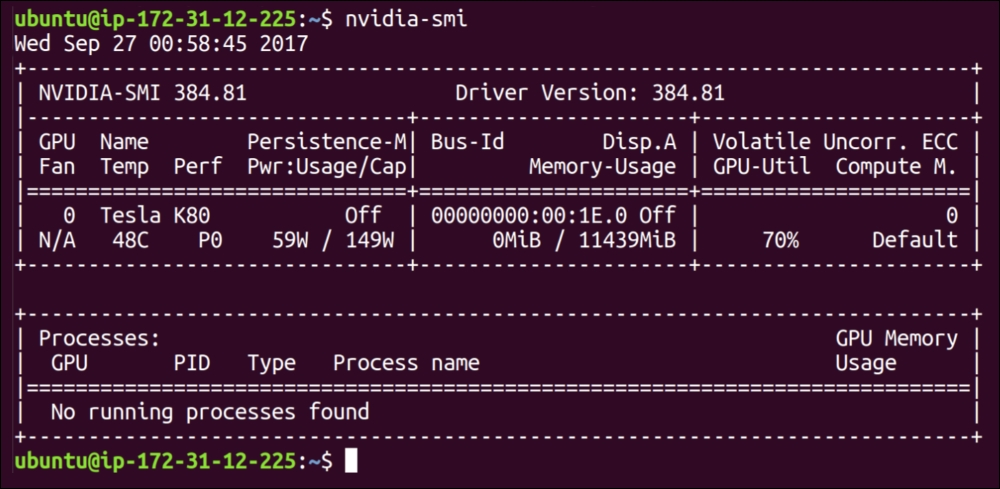Figure 4: Outcome of the nvidia-smi command

#### Installing NVIDIA CUDA

To use TensorFlow with NVIDIA GPUs, CUDA® Toolkit 8.0, and associated NVIDIA drivers with CUDA toolkit 8+ are required to be installed. The CUDA toolkit includes:

• GPU-accelerated libraries such as cuFFT for Fast Fourier Transforms (FFT)

• cuBLAS for Basic Linear Algebra Subroutines (BLAS)

• cuSPARSE for sparse matrix routines

• cuSOLVER for dense and sparse direct solvers

• cuRAND for random number generation, NPP for image, and video processing primitives

• nvGRAPH for NVIDIA Graph Analytics Library

• Thrust for template parallel algorithms and data structures and a dedicated CUDA math library

https://developer.nvidia.com/cuda-downloads using the `wget` command on Ubuntu as follows:

```\$ wget https://developer.nvidia.com/compute/cuda/8.0/Prod2/local_installers/cuda_8.0.61_375.26_linux-run
\$ sudo chmod +x cuda_8.0.61_375.26_linux.run
\$ ./ cuda_8.0.61_375.26_linux.run --driver --silent
\$ ./ cuda_8.0.61_375.26_linux.run --toolkit --silent
\$ ./ cuda_8.0.61_375.26_linux.run --samples –silent
```

Also, ensure that you have added the CUDA installation path to the `LD_LIBRARY_PATH` environment variable as follows:

```\$ echo 'export LD_LIBRARY_PATH="\$LD_LIBRARY_PATH:/usr/local/cuda/lib64:/usr/local/cuda/extras/CUPTI/lib64"' >> ~/.bashrc
\$ echo 'export CUDA_HOME=/usr/local/cuda' >> ~/.bashrc
\$ source ~/.bashrc
```

#### Installing NVIDIA cuDNN v5.1+

Once the CUDA Toolkit is installed, you should download the cuDNN v5.1 library from for Linux and once downloaded, uncompress the files and copy them into the CUDA Toolkit directory (assumed here to be in /usr/local/cuda/):

```\$ cd /usr/local
\$sudo mkdir cuda
\$ sudo tar –xvzf cudnn-8.0-linux-x64-v6.0.tgz
\$ cp cuda/lib64/* /usr/local/cuda/lib64/
\$ cp cuda/include/cudnn.h /usr/local/cuda/include/
```

Note that to install the cuDNN v5.1 library, you must need to register for the Accelerated Computing Developer Program at https://developer.nvidia.com/accelerated-computing-developer. Now when you have installed the cuDNN v5.1 library, ensure that you create the `CUDA_HOME` environment variable.

#### Installing the libcupti-dev Library

Lastly, you need to have the libcupti-dev library installed on your machine. This is the NVIDIA CUDA that provides advanced profiling support. To install this library, issue the following command:

```\$ sudo apt-get install libcupti-dev
```

#### Installing TensorFlow

Refer to the following section for more step-by-step guidelines on how to install the latest version of TensorFlow for the CPU only and GPU supports with NVIDIA cuDNN and CUDA computing capability. You can install TensorFlow on your machine in a number of ways, such as using virtualenv, pip, Docker, and Anaconda. However, using Docker and Anaconda is a bit advanced and this is why we have decided to use pip and virtualenv instead.

### Note

Interested readers can try using Docker and Anaconda from https://www.tensorflow.org/install/.

##### Installing TensorFlow with native pip

If steps 1 to 6 are completed, install TensorFlow by invoking one of the following commands. For Python 2.7 and, of course, with only CPU support:

```\$ pip install tensorflow
# For Python 3.x and of course with only CPU support:
\$ pip3 install tensorflow
# For Python 2.7 and of course with GPU support:
\$ pip install tensorflow-gpu
# For Python 3.x and of course with GPU support:
\$ pip3 install tensorflow-gpu
```

If step 3 failed somehow, install the latest version of TensorFlow by issuing a command manually:

```\$ sudo pip install --upgrade TF_PYTHON_URL
#For Python 3.x, use the following command:
\$ sudo pip3 install --upgrade TF_PYTHON_URL
```

For both cases, `TF_PYTHON_URL` signifies the URL of the TensorFlow Python package presented at https://www.tensorflow.org/install/install_linux#the_url_of_the_tensorflow_python_package.

For example, to install the latest version with CPU-only support (at the time of writing v1.1.0), use the following command:

```\$ sudo pip3 install --upgrade https://storage.googleapis.com/tensorflow/linux/cpu/tensorflow-1.1.0-cp34-cp34m-linux_x86_64.wh
l
```
##### Installing with virtualenv

We assume that you already have Python 2+ (or 3+) and pip (or pip3) installed on your system. If so, follow these steps to install TensorFlow:

1. Create a virtualenv environment as follows:

```\$ virtualenv --system-site-packages targetDirectory
```

The `targetDirectory `signifies the root of the `virtualenv` tree. By default, it is `~/tensorflow` (however, you may choose any directory).

2. Activate virtualenv environment as follows:

```\$ source ~/tensorflow/bin/activate # bash, sh, ksh, or zsh
\$ source ~/tensorflow/bin/activate.csh  # csh or tcsh
```

If the command succeeds in step 2, then you should see the following on your terminal:

`  (tensorflow)\$`
3. Installing TensorFlow.

Follow one of the following commands to install TensorFlow in the active virtualenv environment. For Python 2.7 with CPU-only support, use the following command:

``` (tensorflow)\$ pip install --upgrade tensorflow
#For Python 3.x with CPU support, use the following command:
#For Python 2.7 with GPU support, use the following command:
#For Python 3.x with GPU support, use the following command:
```

If the preceding command succeeds, skip step 5. If the preceding command fails, perform step 5. Moreover, if step 3 failed somehow, try to install TensorFlow in the active virtualenv environment by issuing a command of the following format:

```#For python 2.7 (select appropriate URL with CPU or GPU support):
#For python 3.x (select appropriate URL with CPU or GPU support):
```
4. Validate the installation.

To validate the installation in step 3, you must activate the virtual environment. If the virtualenv environment is not currently active, issue one of the following commands:

```\$ source ~/tensorflow/bin/activate  # bash, sh, ksh, or zsh
\$ source ~/tensorflow/bin/activate.csh  # csh or tcsh
```
5. Uninstalling TensorFlow

To uninstall TensorFlow, simply remove the tree you created. For example:

```\$ rm -r targetDirectory
```

Finally, if you want to control which devices are visible to TensorFlow manually, you should set the `CUDA_VISIBLE_DEVICES`. For example, the following command can be used to force the use of only GPU 0:

```\$ CUDA_VISIBLE_DEVICES=0 python
```

### Installing TensorFlow from Source

The pip installation can cause problems using TensorBoard (this will be discussed later in this lesson). For this reason, I suggest you build TensorFlow directly from the source. The steps are described as follows.

### Note

Follow the instructions and guidelines on how to install Bazel on your platform at http://bazel.io/docs/install.html.

At first, clone the entire TensorFlow repository as follows:

```\$git clone --recurse-submodules https://github.com/tensorflow/tensorflow
```

Then it's time to install Bazel, which is a tool that automates software builds and tests. Also, for building TensorFlow from source, Bazel build system must be installed on your machine. For this, issue the following command:

```\$ sudo apt-get install software-properties-common swig
\$ sudo apt-get update \$ sudo apt-get install oracle-java8-installer
\$ echo "deb http://storage.googleapis.com/bazel-apt stable jdk1.8" | sudo tee /etc/apt/sources.list.d/bazel.list
\$ sudo apt-get update
\$ sudo apt-get install bazel
```

Then run the Bazel installer by issuing the following command:

```\$ chmod +x bazel-version-installer-os.sh
\$ ./bazel-version-installer-os.sh –-user
```

Moreover, you might need some Python dependencies such as `python-numpy`, `swig`, and `python-dev`. Now, issue the following command for doing so:

```\$ sudo apt-get install python-numpy swig python-dev
```

Now it's time to configure the installation (GPU or CPU). Let's do it by executing the following command:

```\$ ./configure
```

Then create your TensorFlow package using `bazel`:

```\$ bazel build -c opt //tensorflow/tools/pip_package:
\$ build_pip_package
```

However, to build with the GPU support, issue the following command:

```\$ bazel build -c opt --config=cuda //tensorflow/tools/pip_package:build_pip_package
```

Finally, install TensorFlow. Here I have listed, as per the Python version:

• For Python 2.7:

```\$ sudo pip install --upgrade /tmp/tensorflow_pkg/tensorflow-1.1.0-*.whl
```
• For Python 3.4:

```\$ sudo pip3 install --upgrade /tmp/tensorflow_pkg/tensorflow-1.1.0-*.whl
```

We start with the popular TensorFlow alias `tf`. Open a Python terminal (just type `python` or `python3` on terminal) and issue the following lines of code:

`>>> import tensorflow as tf`

If your favourite Python interpreter doesn't complain, then you're ready to start using TensorFlow!

```>>> hello = tf.constant("Hello, TensorFlow!")
>>> sess=tf.Session()```

Now to verify your installation just type the following:

`>>> print sess.run(hello)`

If the installation is OK, you'll see the following output:

`Hello, TensorFlow!`

## TensorFlow Computational Graph

When thinking of execution of a TensorFlow program we should be familiar with a graph creation and a session execution. Basically the first one is for building the model and the second one is for feeding the data in and getting the results. An interesting thing is that TensorFlow does each and everything on the C++ engine, which means even a little multiplication or addition is not executed on Python but Python is just a wrapper. Fundamentally, TensorFlow C++ engine consists of following two things:

• Efficient implementations for operations like convolution, max pool, sigmoid, and so on.

• Derivatives of forwarding mode operation.

When we/you're performing a little complex operation with TensorFlow, for example training a linear regression, TensorFlow internally represents its computation using a dataflow graph. The graph is called a computational graph, which is a directed graph consisting of the following:

• A set of nodes, each one representing an operation

• A set of directed arcs, each one representing the data on which the operations are performed.

TensorFlow has two types of edges:

• Normal: They carry the data structures between the nodes. The output of one operation from one node, becomes input for another operation. The edge connecting two nodes carries the values.

• Special: This edge doesn't carry values, but only represents a control dependency between two nodes, say X and Y. It means that the node Y will be executed only if the operation in X is executed already, but before the relationship between operations on the data.

The TensorFlow implementation defines control dependencies to enforce orderings between otherwise independent operations as a way of controlling the peak memory usage.

A computational graph is basically like a dataflow graph. Figure 5 shows a computational graph for a simple computation like z=d×c=(a+b) ×c: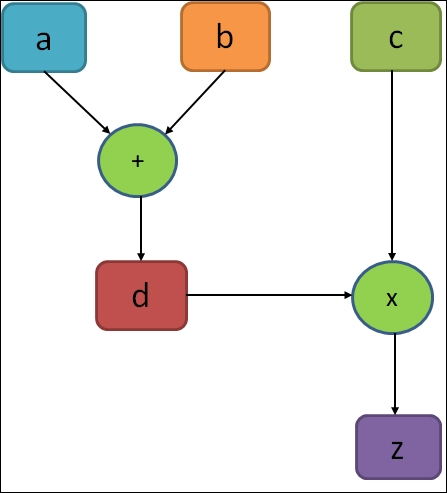Figure 5: A very simple execution graph that computes a simple equation

In the preceding figure, the circles in the graph indicate the operations, while rectangles indicate a data computational graph. As stated earlier, a TensorFlow graph contains the following:

• A set of tf.Operation objects: This is used to represent units of computation to be performed

• A tf.Tensor object: This is used to represent units of data that control the dataflow between operations

Using TensorFlow, it is also possible to perform a deferred execution. To give an idea, once you have composed a highly compositional expression during the building phase of the computational graph, you can still evaluate them in the running session phase. Technically saying TensorFlow schedules the job and executes on time in an efficient manner. For example, parallel execution of independent parts of the code using the GPU is shown in figure 6.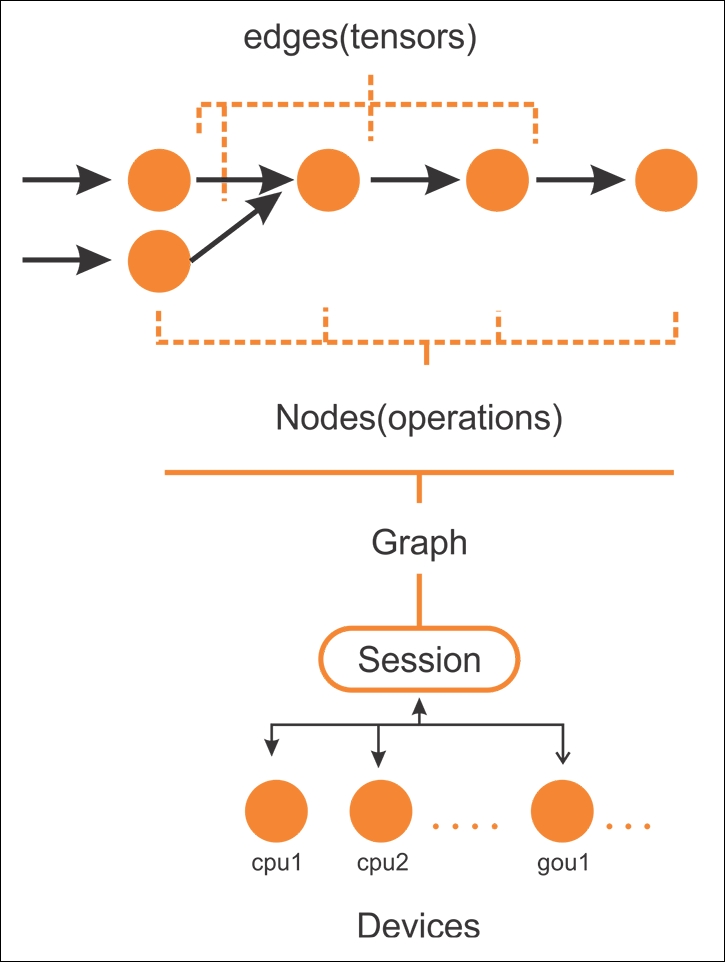Figure 6: Edges and nodes in TensorFlow graph to be executed under a session on devices such as CPUs or GPUs

After a computational graph is created, TensorFlow needs to have an active session to be executed by multiple CPUs (and GPUs if available) in a distributed way. In general, you really don't need to specify whether to use a CPU or a GPU explicitly, since TensorFlow can choose and use which one is to be used. By default, a GPU will be picked for as many operations as possible; otherwise, a CPU will be used. So in a broad view, here are the main components of TensorFlow:

• Variables: Used to contain values for the weights and bias between TensorFlow sessions.

• Tensors: A set of values that pass in between nodes.

• Placeholders: Is used to send data between the program and the TensorFlow graph.

• Session: When a session is started, TensorFlow automatically calculates gradients for all the operations in the graph and use them in a chain rule. In fact, a session is invoked when the graph is to be executed.

Don't worry much, each of the preceding components will be discussed in later sections. Technically saying, the program you will be writing can be considered as a client. The client is then used to create the execution graph in C/C++ or Python symbolically, and then your code can ask TensorFlow to execute this graph. See details in the following figure: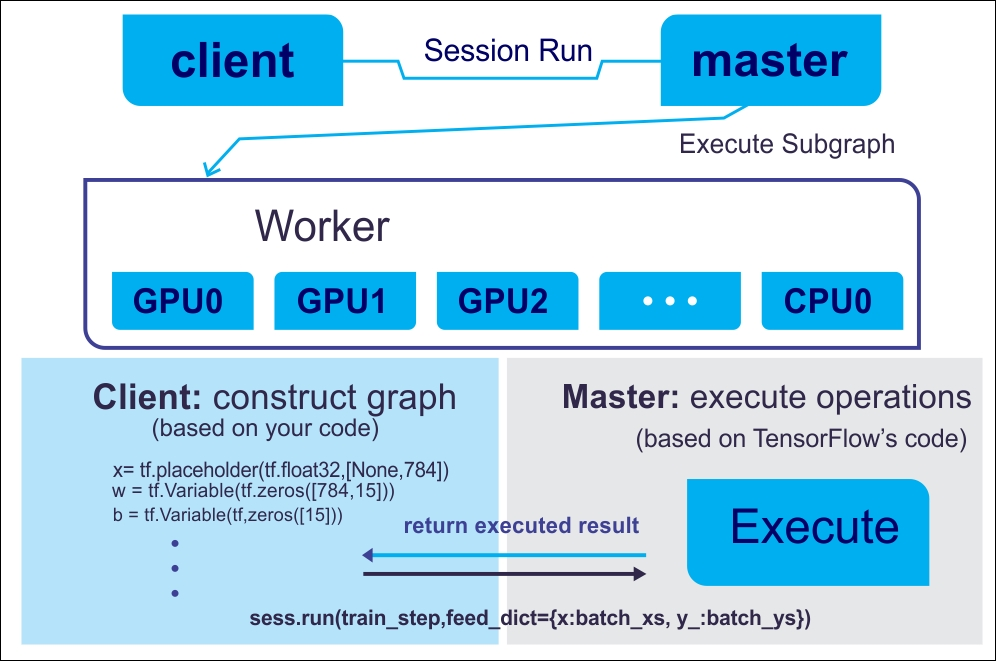Figure 7: Using a client–master architecture for executing TensorFlow graph

A computational graph helps to distribute the work load across multiple computing nodes having a CPU or a GPU. This way, a neural network can further be equaled to a composite function where each layer (input, hidden or output layer) can be represented as a function. Now to understand the operations performed on the tensors, knowing a good workaround about TensorFlow programming model is a mandate. The next section explains the role of the computational graph to implement a neural network.

## TensorFlow Programming Model

The TensorFlow programming model signifies how to structure your predictive models. A TensorFlow program is generally divided into four phases once you have imported TensorFlow library for associated resources:

• Construction of the computational graph that involves some operations on tensors (we will see what is a tensor soon)

• Create a session

• Running a session, that is performed for the operations defined in the graph

• Computation for data collection and analysis

These main steps define the programming model in TensorFlow. Consider the following example, in which we want to multiply two numbers:

```import tensorflow as tf
x = tf.constant(8)
y = tf.constant(9)
z = tf.multiply(x, y)
sess = tf.Session()
out_z = sess.run(z)
Finally, close the TensorFlow session when you're done:
sess.close()print('The multiplicaiton of x and y: %d' % out_z)```

The preceding code segment can be represented by the following figure: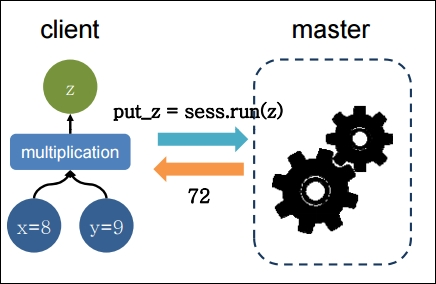Figure 8: A simple multiplication executed and returned the product on client-master architecture

To make the preceding program more efficient, TensorFlow also allows you to exchange data in your graph variables through placeholders (to be discussed later). Now imagine the following code segment that does the same but in a more efficient way:

```# Import tensorflow
import tensorflow as tf
# Build a graph and create session passing the graph:
with tf.Session() as sess:
x = tf.placeholder(tf.float32, name="x")
y = tf.placeholder(tf.float32, name="y")
z = tf.multiply(x,y)
# Put the values 8,9 on the placeholders x,y and execute the graph
z_output = sess.run(z,feed_dict={x: 8, y:9})
# Finally, close the TensorFlow session when you're done:
sess.close()
print(z_output)```

TensorFlow is not necessary to multiply two numbers; also the number of lines of the code for this simple operation is so many. However, the example wants to clarify how to structure any code, from the simplest as in this instance, to the most complex. Furthermore, the example also contains some basic instructions that we will find in all the other examples given in the course of this book.

### Note

We will demonstrate most of the examples in this book with Python 3.x compatible. However, a few examples will be given using Python 2.7.x too.

This single import in the first line helps to import the TensorFlow for your command that can be instantiated with `tf as stated earlier. Then the `TensorFlow operator will then be expressed by `tf` and the dot '`.`' and by the name of the operator to use. In the next line, we construct the object `session`, by means of the instruction `tf.Session()`:

`with tf.Session() as sess:`

### Note

The session object (that is, sess) encapsulates the environment for the TensorFlow so that all the operation objects are executed, and Tensor objects are evaluated. We will see them in upcoming sections.

This object contains the computation graph, which as we said earlier, are the calculations to be carried out.

The following two lines define variables x and y, using the notion of placeholder. Through a placeholder you may define both an input (such as the variable x of our example) and an output variable (such as the variable y):

```x = tf.placeholder(tf.float32, name='x')
y = tf.placeholder(tf.float32, name='y')```

Placeholder provides an interface between the elements of the graph and the computational data of the problem, it allows us to create our operations and build our computation graph, without needing the data, but only a reference to it.

To define a data or tensor (soon I will introduce you to the concept of tensor) via the placeholder function, three arguments are required:

• Data type: Is the type of element in the tensor to be fed.

• Shape: Of the placeholder–that is, shape of the tensor to be fed (optional). If the shape is not specified, you can feed a tensor of any shape.

• Name: Very useful for debugging and code analysis purposes, but it is optional.

### Note

For more, refer to https://www.tensorflow.org/api_docs/python/tf/Tensor.

So, we may introduce the model that we want to compute with two arguments, the placeholder and the constant that are previously defined. Next, we define the computational model.

The following statement, inside the session, builds the data structures of the `x` product with `y`, and the subsequent assignment of the result of the operation to the placeholder `z`. Then it goes as follows:

`  z = tf.multiply(x, y)`

Now since the result is already held by the placeholder `z`, we execute the graph, through the `sess.run`statement. Here we feed two values to patch a tensor into a graph node. It temporarily replaces the output of an operation with a tensor value (more in upcoming sections):

`z_output = sess.run(z,feed_dict={x: 8, y:9})`

Then we close the TensorFlow session when we're done:

`sess.close()`

In the final instruction, we print out the result:

`     print(z_output)`

This essentially prints output 72.0.

## Data Model in TensorFlow

The data model in TensorFlow is represented by tensors. Without using complex mathematical definitions, we can say that a tensor (in TensorFlow) identifies a multidimensional numerical array. But we will see more details on tensor in the next sub-section.

### Tensors

Let's see a formal definition of tensors from Wikipedia (https://en.wikipedia.org/wiki/Tensor) as follows:

"Tensors are geometric objects that describe linear relations between geometric vectors, scalars, and other tensors. Elementary examples of such relations include the dot product, the cross product, and linear maps. Geometric vectors, often used in physics and engineering applications, and scalars themselves are also tensors."

This data structure is characterized by three parameters: Rank, Shape, and Type, as shown in the following figure: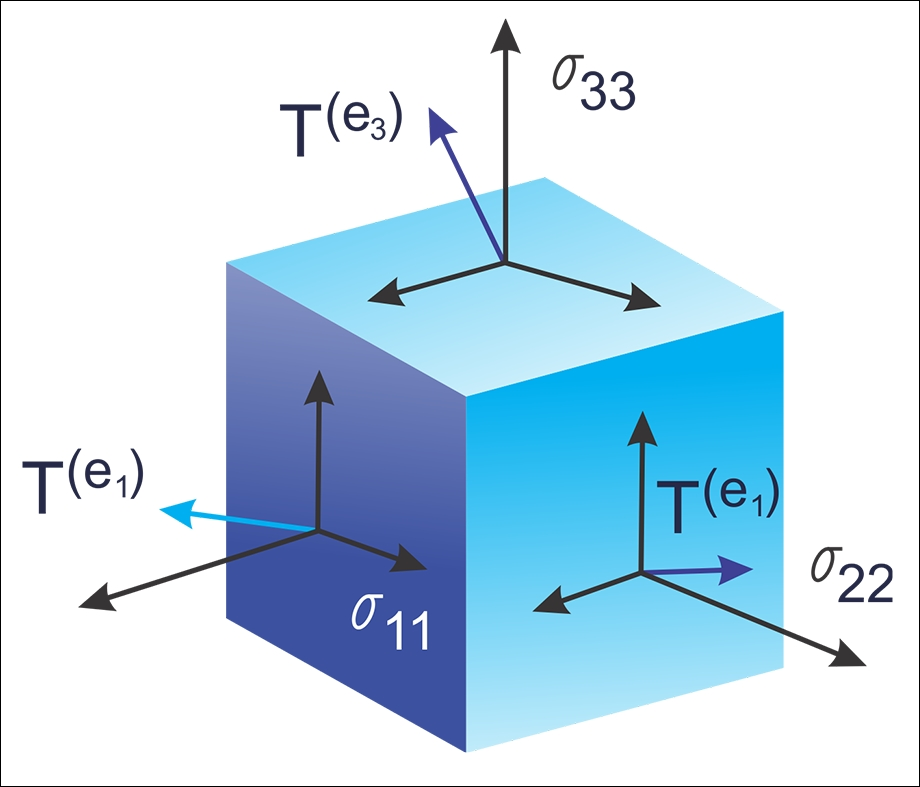Figure 9: Tensors are nothing but geometrics objects having shape, rank, and type used to hold multidimensional arrays

A tensor thus can be thought of as a generalization of a matrix that specifies an element by an arbitrary number of indices. While practically used, the syntax for tensors is even more or less like nested vectors.

### Note

Tensors just define the type of this value and the means by which this value should be calculated during the session. Therefore, essentially, they do not represent or hold any value produced by an operation.

A few people love to compare NumPy versus TensorFlow comparison; however, in reality, TensorFlow and NumPy are quite similar in a sense that both are N-d array libraries!

Well, it's true that NumPy has the n–dimensional array support, but it doesn't offer methods to create tensor functions and automatically compute derivatives (+ no GPU support). The following table can be seen as a short and one-to-one comparison that could make some sense of such comparisons: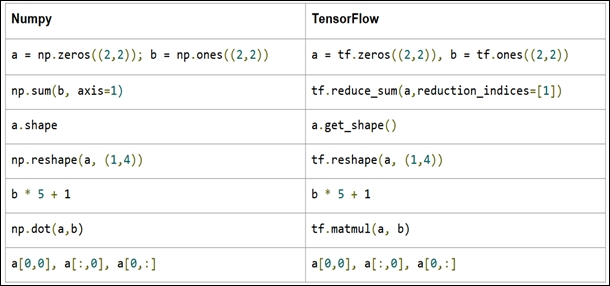Figure 10: NumPy versus TensorFlow

Now let's see an alternative way of creating tensors before they could be fed (we will see other feeding mechanisms later on) by the TensorFlow graph:

```>>> X = [[2.0, 4.0],
[6.0, 8.0]]
>>> Y = np.array([[2.0, 4.0],
[6.0, 6.0]], dtype=np.float32)
>>> Z = tf.constant([[2.0, 4.0],
[6.0, 8.0]])```

Here `X` is a list, `Y` is an n-dimensional array from the NumPy library, and `Z` is itself the TensorFlow's Tensor object. Now let's see their types:

```>>> print(type(X))
>>> print(type(Y))
>>> print(type(Z))
#Output
<class 'list'>
<class 'numpy.ndarray'>
<class 'tensorflow.python.framework.ops.Tensor'>```

Well, their types are printed correctly. However, a more convenient function that we're formally dealing with tensors, as opposed to the other types is `tf.convert_to_tensor()` function as follows:

`t1 = tf.convert_to_tensor(X, dtype=tf.float32)t2 = tf.convert_to_tensor(Z, dtype=tf.float32)t3 = tf.convert_to_tensor(Z, dtype=tf.float32)`

Now let's see their type using the following lines:

```>>> print(type(t1))
>>> print(type(t2))
>>> print(type(t3))
#Output:
<class 'tensorflow.python.framework.ops.Tensor'>
<class 'tensorflow.python.framework.ops.Tensor'>
<class 'tensorflow.python.framework.ops.Tensor'>```

Fantastic! I think up to now it's enough discussion already carried out on tensors, so now we can think about the structure that is characterized by the term rank.

### Rank

Each tensor is described by a unit of dimensionality called rank. It identifies the number of dimensions of the tensor, for this reason, a rank is known as order or n–dimensions of a tensor. A rank zero tensor is a scalar, a rank one tensor id a vector, while a rank two tensor is a matrix. The following code defines a TensorFlow scalar, a `vector`, a `matrix`, and a `cube_matrix`, in the next example we will show how the rank works:

```import tensorflow as tf
scalar = tf.constant(100)
vector = tf.constant([1,2,3,4,5])
matrix = tf.constant([[1,2,3],[4,5,6]])
cube_matrix = tf.constant([[,,],[,,],[,,]])
print(scalar.get_shape())
print(vector.get_shape())
print(matrix.get_shape())
print(cube_matrix.get_shape())```

The results are printed here:

```>>>
()
(5,)
(2, 3)
(3, 3, 1)
>>>```

### Shape

The shape of a tensor is the number of rows and columns it has. Now we will see how to relate the shape to a rank of a tensor:

```>>scalar1.get_shape()
TensorShape([])
>>vector1.get_shape()
TensorShape([Dimension(5)])
>>matrix1.get_shape()
TensorShape([Dimension(2), Dimension(3)])
>>cube1.get_shape()
TensorShape([Dimension(3), Dimension(3), Dimension(1)])```

### Data Type

In addition to rank and shape, tensors have a data type. The following is the list of the data types: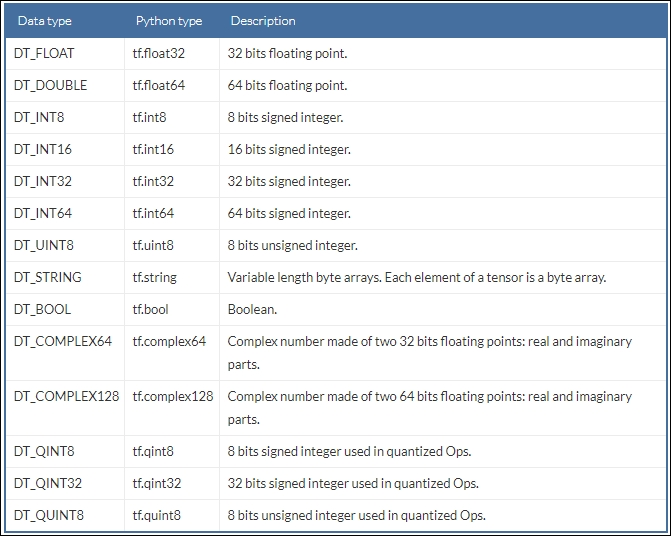We believe the preceding table is self-explanatory hence we did not provide detailed discussion on the preceding data types. Now the TensorFlow APIs are implemented to manage data to and from NumPy arrays. Thus, to build a tensor with a constant value, pass a NumPy array to the `tf.constant()` operator, and the result will be a TensorFlow tensor with that value:

```import tensorflow as tf
import numpy as np
tensor_1d = np.array([1,2,3,4,5,6,7,8,9,10])
tensor_1d = tf.constant(tensor_1d)
with tf.Session() as sess:
print (tensor_1d.get_shape())
print sess.run(tensor_1d)
# Finally, close the TensorFlow session when you're done
sess.close()```

Running the example, we obtain:

```>>>
(10,)
[ 1  2  3  4  5  6  7  8  9 10]```

To build a tensor, with variable values, use a `NumPy` array and pass it to the `tf.Variable `constructor, the result will be a TensorFlow variable tensor with that initial value:

```import tensorflow as tf
import numpy as np
tensor_2d = np.array([(1,2,3),(4,5,6),(7,8,9)])
tensor_2d = tf.Variable(tensor_2d)
with tf.Session() as sess:
sess.run(tf.global_variables_initializer())
print (tensor_2d.get_shape())
print sess.run(tensor_2d)
# Finally, close the TensorFlow session when you're done
sess.close()```

The result is:

```>>>
(3, 3)
[[1 2 3]
[4 5 6]
[7 8 9]]```

For ease of use in interactive Python environments, we can use the `InteractiveSession` class, and then use that session for all `Tensor.eval() `and `Operation.run() `calls:

```import tensorflow as tf
import numpy as np

interactive_session = tf.InteractiveSession()
tensor = np.array([1,2,3,4,5])
tensor = tf.constant(tensor)
print(tensor.eval())

interactive_session.close()```

### Note

`tf.InteractiveSession()` is just a convenient syntactic sugar for keeping a default session open in IPython.

The result is:

```>>>
[1 2 3 4 5]```

This can be easier in an interactive setting, such as the shell or an IPython notebook, when it's tedious to pass around a session object everywhere.

### Note

The IPython Notebook is now known as the Jupyter Notebook. It is an interactive computational environment, in which you can combine code execution, rich text, mathematics, plots and rich media. For more information, interested readers should refer to the web page at https://ipython.org/notebook.html.

Another way to define a tensor is using the TensorFlow statement `tf.convert_to_tensor`:

```import tensorflow as tf
import numpy as np
tensor_3d = np.array([[[0, 1, 2], [3, 4, 5], [6, 7, 8]],
[[9, 10, 11], [12, 13, 14], [15, 16, 17]],
[[18, 19, 20], [21, 22, 23], [24, 25, 26]]])
tensor_3d = tf.convert_to_tensor(tensor_3d, dtype=tf.float64)
with tf.Session() as sess:
print(tensor_3d.get_shape())
print(sess.run(tensor_3d))
# Finally, close the TensorFlow session when you're done
sess.close()
>>>
(3, 3, 3)
[[[  0.   1.   2.]
[  3.   4.   5.]
[  6.   7.   8.]]
[[  9.  10.  11.]
[ 12.  13.  14.]
[ 15.  16.  17.]]
[[ 18.  19.  20.]
[ 21.  22.  23.]
[ 24.  25.  26.]]]```

### Variables

Variables are TensorFlow objects to hold and update parameters. A variable must be initialized; also you can save and restore it to analyze your code. Variables are created by using the `tf.Variable() `statement. In the following example, we want to count the numbers from 1 to 10, but let's import TensorFlow first:

`import tensorflow as tf`

We created a variable that will be initialized to the scalar value `0`:

`value = tf.Variable(0, name="value")`

The `assign() `and `add()`operators are just nodes of the computation graph, so they do not execute the assignment until the run of the session:

```one = tf.constant(1)
update_value = tf.assign(value, new_value)
initialize_var = tf.global_variables_initializer()```

We can instantiate the computation graph:

```with tf.Session() as sess:
sess.run(initialize_var)
print(sess.run(value))
for _ in range(5):
sess.run(update_value)
print(sess.run(value))
# Finally, close the TensorFlow session when you're done:
sess.close()```

Let's recall that a tensor object is a symbolic handle to the result of an operation, but it does not actually hold the values of the operation's output:

```>>>
0
1
2
3
4
5```

### Fetches

To fetch the outputs of operations, execute the graph by calling `run()` on the session object and pass in the tensors to retrieve. Except fetching the single tensor node, you can also fetch multiple tensors. In the following example, the sum and multiply tensors are fetched together, using the `run()` call:

```import tensorflow as tf

constant_A = tf.constant([100.0])
constant_B = tf.constant([300.0])
constant_C = tf.constant([3.0])

mul_ = tf.multiply(constant_A,constant_C)

with tf.Session() as sess:
result = sess.run([sum_,mul_])
print(result)

# Finally, close the TensorFlow session when you're done:
sess.close()```

The output is as follows:

```>>>
[array(400.],dtype=float32),array([ 300.],dtype=float32)]```

All the ops needed to produce the values of the requested tensors are run once (not once per requested tensor).

### Feeds and Placeholders

There are four methods of getting data into a TensorFlow program (see details at https://www.tensorflow.org/api_guides/python/reading_data):

• The Dataset API: This enables you to build complex input pipelines from simple and reusable pieces from distributed file systems and perform complex operations. Using the Dataset API is recommended while dealing with large amounts of data in different data formats. The Dataset API introduces two new abstractions to TensorFlow for creating feedable dataset using either `tf.contrib.data.Dataset` (by creating a source or applying a transformation operations) or using a `tf.contrib.data.Iterator`.

• Feeding: Allows us to inject data into any Tensor in a computation graph.

• Reading from files: We can develop an input pipeline using Python's built-in mechanism for reading data from data files at the beginning of a TensorFlow graph.

• Preloaded data: For small datasets, we can use either constants or variables in the TensorFlow graph for holding all the data.

In this section, we will see an example of the feeding mechanism only. For the other methods, we will see them in upcoming lesson. TensorFlow provides the feed mechanism that allows us inject data into any tensor in a computation graph. You can provide the feed data through the `feed_dict` argument to a `run()` or `eval()`invoke that initiates the computation.

### Note

Feeding using the `feed_dict` argument is the least efficient way to feed data into a TensorFlow execution graph and should only be used for small experiments needing small datasets. It can also be used for debugging.

We can also replace any tensor with feed data (that is variables and constants), the best practice is to use a TensorFlow placeholder node using `tf.placeholder()` invocation. A placeholder exists exclusively to serve as the target of feeds. An empty placeholder is not initialized so it does not contain any data. Therefore, it will always generate an error if it is executed without a feed, so you won't forget to feed it.

The following example shows how to feed data to build a random 2×3 matrix:

```import tensorflow as tf
import numpy as np

a = 3
b = 2
x = tf.placeholder(tf.float32,shape=(a,b))

data = np.random.rand(a,b)
sess = tf.Session()
print sess.run(y,feed_dict={x:data})

# Finally, close the TensorFlow session when you're done:
sess.close()```

The output is:

```>>>
[[ 1.78602004  1.64606333]
[ 1.03966308  0.99269408]
[ 0.98822606  1.50157797]]
>>>```

## TensorBoard

TensorFlow includes functions to debug and optimize programs in a visualization tool called TensorBoard. Using TensorBoard, you can observe different types of statistics concerning the parameters and details of any part of the graph computing graphically.

Moreover, while doing predictive modeling using the complex deep neural network, the graph can be complex and confusing. Thus to make it easier to understand, debug, and optimize TensorFlow programs, you can use TensorBoard to visualize your TensorFlow graph, plot quantitative metrics about the execution of your graph, and show additional data such as images that pass through it.

Therefore, the TensorBoard can be thought of as a framework designed for analysis and debugging of predictive models. TensorBoard uses the so-called summaries to view the parameters of the model: once a TensorFlow code is executed, we can call TensorBoard to view summaries in a GUI.

### How Does TensorBoard Work?

As explained previously, TensorFlow uses the computation graph to execute an application, where each node represents an operation and the arcs are the data between operations.

The main idea in TensorBoard is to associate the so-called summary with nodes (operations) of the graph. Upon running the code, the summary operations will serialize the data of the node that is associated with it and output the data into a file that can be read by TensorBoard. Then TensorBoard can be run and visualize the summarized operations. The workflow when using TensorBoard is:

• Attach summary ops to the nodes you are interested in examining

• Start running your graph as you normally would

• Additionally, run the summary ops

• When the code is done running, run TensorBoard to visualize the summary outputs

If you type `\$ which tensorboard` in your terminal, it should exist if you installed with `pip`:

```[email protected]:~\$ which tensorboard
/usr/local/bin/tensorboard
```

You need to give it a log directory, so you are in the directory where you ran your graph; you can launch it from your terminal with something like:

```tensorboard --logdir .
```

Then open your favorite web browser and type in `localhost:6006` to connect. When TensorBoard is fully configured, this can be accessed by issuing the following command:

```\$ tensorboard –logdir=<trace_file_name>
```

Now you simply need to access the local port `6006` from the browser `http://localhost:6006/`. Then it should look like this: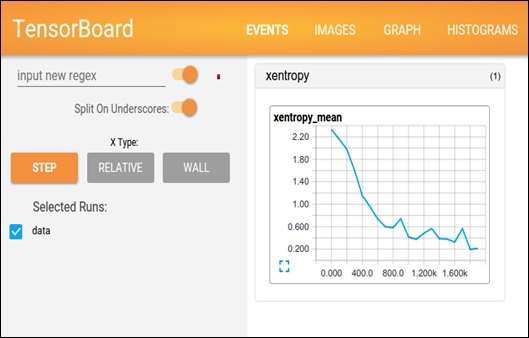Figure 11: Using TensorBoard on browser

Is this already too much? Don't worry, in the last section, we'll combine all the ideas previously explained to build a single input neuron model and to analyze it with TensorBoard.

## Getting Started with TensorFlow – Linear Regression and Beyond

In this example, we will take a closer look at TensorFlow's and TensorBoard's main concepts and try to do some basic operations to get you started. The model we want to implement simulates the linear regression.

In the statistics and machine learning realm, linear regression is a technique frequently used to measure the relationship between variables. This is also a quite simple but effective algorithm that can be used in predictive modeling too. Linear regression models the relationship between a dependent variable yi, an interdependent variable xi, and a random term b. This can be seen as follows: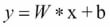Now to conceptualize the preceding equation, I am going to write a simple Python program for creating data into a 2D space. Then I will use TensorFlow to look for the line that best fits in the data points:

```# Import libraries (Numpy, matplotlib)
import numpy as np
import matplotlib.pyplot as plot

# Create 1000 points following a function y=0.1 * x + 0.4 (i.e. y \= W * x + b) with some normal random distribution:

num_points = 1000
vectors_set = []
for i in range(num_points):
W = 0.1 # W
b = 0.4 # b
x1 = np.random.normal(0.0, 1.0)
nd = np.random.normal(0.0, 0.05)
y1 = W * x1 + b

# Add some impurity with some normal distribution -i.e. nd:
y1 = y1+nd

# Append them and create a combined vector set:
vectors_set.append([x1, y1])

# Separate the data point across axises:
x_data = [v for v in vectors_set]
y_data = [v for v in vectors_set]

# Plot and show the data points in a 2D space
plt.plot(x_data, y_data, 'r*', label='Original data')
plt.legend()
plt.show()```

If your compiler does not make any complaints, you should observe the following graph: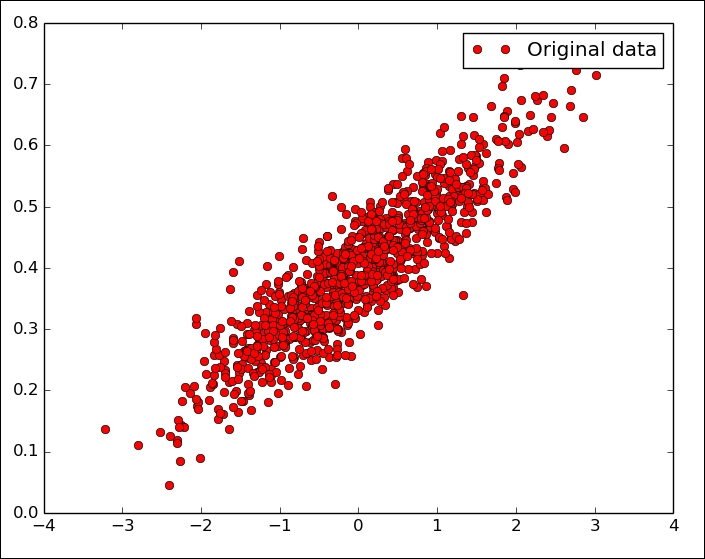Figure 12: Randomly generated (but original) data

Well, so far we have just created a few data points without any associated model that could be executed through TensorFlow. So the next step is to create a linear regression model to be able to obtain the output values `y` that is estimated from the input data points–that is, `x_data`. In this context, we have only two associated parameters–that is, `W` and `b`. Now the objective is to create a graph that allows finding the values for these two parameters based on the input data `x_data` by adjusting them to `y_data`–that is, optimization problem.

So the target function in our case would be as follows: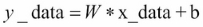If you recall, we defined W = 0.1 and b = 0.4 while creating the data points in the 2D space. Now TensorFlow has to optimize these two values so that `W` tends to 0.1 and `b` to 0.4, but without knowing any optimization function, TensorFlow does not even know anything.

A standard way to solve such optimization problems is to iterate through each value of the data points and adjust the value of `W` and `b` in order to get a more precise answer on each iteration. Now to realize if the values are really improving, we need to define a cost function that measures how good a certain line is.

In our case, the cost function is the mean squared error that helps find the average of the errors based on the distance function between the real data points and the estimated ones on each iteration. We start by importing the TensorFlow library:

```import tensorflow as tf
W = tf.Variable(tf.random_uniform(, -1.0, 1.0))
b = tf.Variable(tf.zeros())
y = W * x_data + b```

In the preceding code segment, we are generating a random point using a different strategy and storing in variable W. Now let's define a loss function loss=mean [(y−y_data) 2] and this returns a scalar value with the mean of all distances between our data and the model prediction. In terms of TensorFlow convention, the loss function can be expressed as follows:

`loss = tf.reduce_mean(tf.square(y - y_data))`

Without going into further detail, we can use some widely used optimization algorithms such as gradient descent. At a minimal level, the gradient descent is an algorithm that works on a set of given parameters that we already have. It starts with an initial set of parameter values and iteratively moves toward a set of values that minimize the function by taking another parameter called learning rate. This iterative minimization is achieved by taking steps in the negative direction of the function called gradient.

```optimizer = tf.train.GradientDescentOptimizer(0.6)
train = optimizer.minimize(loss)```

Before running this optimization function, we need to initialize all the variables that we have so far. Let's do it using TensorFlow convention as follows:

```init = tf.global_variables_initializer()
sess = tf.Session()
sess.run(init)```

Since we have created a TensorFlow session, we are ready for the iterative process that helps us find the optimal values of `W` and `b`:

```for i in range(16):
sess.run(train)
print(i, sess.run(W), sess.run(b), sess.run(loss))```

You should observe the following output:

```>>>
0 [ 0.18418592] [ 0.47198644] 0.0152888
1 [ 0.08373772] [ 0.38146532] 0.00311204
2 [ 0.10470386] [ 0.39876288] 0.00262051
3 [ 0.10031486] [ 0.39547175] 0.00260051
4 [ 0.10123629] [ 0.39609471] 0.00259969
5 [ 0.1010423] [ 0.39597753] 0.00259966
6 [ 0.10108326] [ 0.3959994] 0.00259966
7 [ 0.10107458] [ 0.39599535] 0.00259966```

Thus you can see the algorithm starts with the initial values of W = 0.18418592 and b = 0.47198644 where the loss is pretty high. Then the algorithm iteratively adjusted the values by minimizing the cost function. In the eighth iteration, all the values tend to our desired values.

Now what if we could plot them? Let's do it by adding the plotting line under the `for` loop as follows: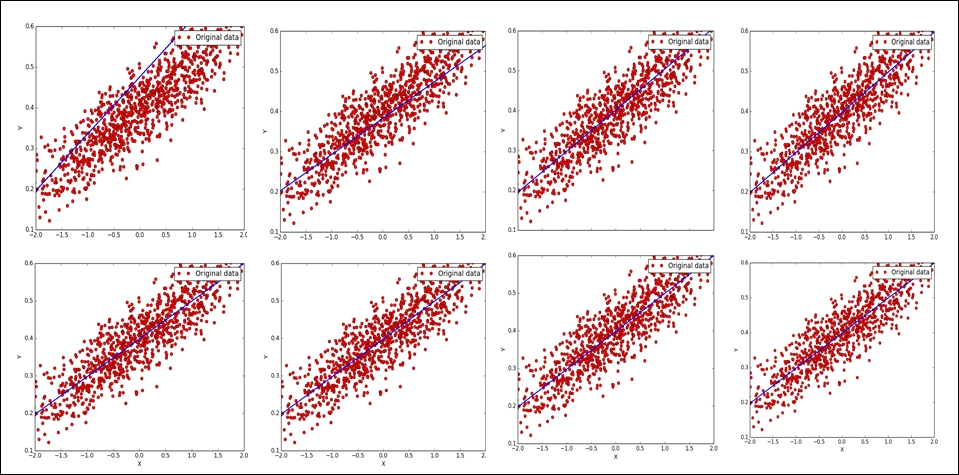Figure 13: Linear regression after eight iteration that optimizes the loss function

Now let's iterate the same up to the 16th iteration:

```>>>
0 [ 0.23306453] [ 0.47967502] 0.0259004
1 [ 0.08183448] [ 0.38200468] 0.00311023
2 [ 0.10253634] [ 0.40177572] 0.00254209
3 [ 0.09969243] [ 0.39778906] 0.0025257
4 [ 0.10008509] [ 0.39859086] 0.00252516
5 [ 0.10003048] [ 0.39842987] 0.00252514
6 [ 0.10003816] [ 0.39846218] 0.00252514
7 [ 0.10003706] [ 0.39845571] 0.00252514
8 [ 0.10003722] [ 0.39845699] 0.00252514
9 [ 0.10003719] [ 0.39845672] 0.00252514
10 [ 0.1000372] [ 0.39845678] 0.00252514
11 [ 0.1000372] [ 0.39845678] 0.00252514
12 [ 0.1000372] [ 0.39845678] 0.00252514
13 [ 0.1000372] [ 0.39845678] 0.00252514
14 [ 0.1000372] [ 0.39845678] 0.00252514
15 [ 0.1000372] [ 0.39845678] 0.00252514```

Much better and we're closer to the optimized values, right? Now, what if we further improve our visual analytics through TensorFlow that help visualize what is happening in these graphs. TensorBoard provides a web page for debugging your graph as well as inspecting the used variables, node, edges, and their corresponding connections.

However, to get the facility of the preceding regression analysis, you need to annotate the preceding graphs with the variables such as loss function, `W`, `b`, `y_data`, `x_data`, and so on. Then you need to generate all the summaries by invoking the function `tf.summary.merge_all()`.

Now, we need to make the following changes to the preceding code. However, it is a good practice to group related nodes on the graph using the `tf.name_scope()` function. Thus, we can use `tf.name_scope()` to organize things on the TensorBoard graph view, but let's give it a better name:

```with tf.name_scope("LinearRegression") as scope:
W = tf.Variable(tf.random_uniform(, -1.0, 1.0), name="Weights")
b = tf.Variable(tf.zeros())y = W * x_data + b```

Then let's annotate the loss function in a similar way, but by giving a suitable name such as `LossFunction`:

```with tf.name_scope("LossFunction") as scope:
loss = tf.reduce_mean(tf.square(y - y_data))```

Let's annotate the loss, weights, and bias that are needed for the TensorBoard:

```loss_summary = tf.summary.scalar("loss", loss)
w_ = tf.summary.histogram("W", W)
b_ = tf.summary.histogram("b", b)```

Well, once you annotate the graph, it's time to configure the summary by merging them:

`merged_op = tf.summary.merge_all()`

Now before running the training (after the initialization), write the summary using the `tf.summary.FileWriter()` API as follows:

`writer_tensorboard = tf.summary.FileWriter('/home/asif/LR/', sess.graph_def)`

Then start the TensorBoard as follows:

```\$ tensorboard –logdir=<trace_file_name>
```

In our case, it could be something like the following:

```\$ tensorboard --logdir=/home/asif/LR/
```

Now let's move to `http://localhost:6006` and on clicking on the GRAPHS tab, you should see the following graph: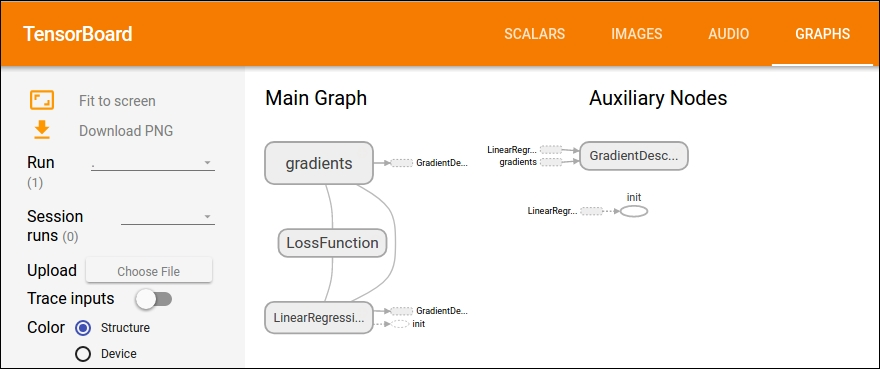Figure 14: Main graph and auxiliary nodes on TensorBoard

### Source Code for the Linear Regression

We reported for the entire source code for the example previously described:

```# Import libraries (Numpy, Tensorflow, matplotlib)
import numpy as np
import matplotlib.pyplot as plot

# Create 1000 points following a function y=0.1 * x + 0.4 (i.e. y = W * x + b) with some normal random distribution:
num_points = 1000
vectors_set = []
for i in range(num_points):
W = 0.1  # W
b = 0.4  # b
x1 = np.random.normal(0.0, 1.0)
nd = np.random.normal(0.0, 0.05)
y1 = W * x1 + b

# Add some impurity with some normal distribution -i.e. nd:y1 = y1 + nd

# Append them and create a combined vector set:
vectors_set.append([x1, y1])

# Separate the data point across axises
x_data = [v for v in vectors_set]
y_data = [v for v in vectors_set]

# Plot and show the data points in a 2D space
plot.plot(x_data, y_data, 'ro', label='Original data')
plot.legend()
plot.show()

import tensorflow as tf

#tf.name_scope organize things on the tensorboard graph view
with tf.name_scope("LinearRegression") as scope:
W = tf.Variable(tf.random_uniform(, -1.0, 1.0), name="Weights")
b = tf.Variable(tf.zeros())
y = W * x_data + b

# Define a loss function that takes into account the distance between the prediction and our dataset
with tf.name_scope("LossFunction") as scope:
loss = tf.reduce_mean(tf.square(y - y_data))

train = optimizer.minimize(loss)

# Annotate loss, weights, and bias (Needed for tensorboard)
loss_summary = tf.summary.scalar("loss", loss)
w_ = tf.summary.histogram("W", W)
b_ = tf.summary.histogram("b", b)

# Merge all the summaries
merged_op = tf.summary.merge_all()

init = tf.global_variables_initializer()
sess = tf.Session()
sess.run(init)

# Writer for TensorBoard  (replace with our preferred location
writer_tensorboard = tf.summary.FileWriter('/ LR/', sess.graph_def)

for i in range(16):
sess.run(train)
print(i, sess.run(W), sess.run(b), sess.run(loss))
plot.plot(x_data, y_data, 'ro', label='Original data')
plot.plot(x_data, sess.run(W)*x_data + sess.run(b))
plot.xlabel('X')
plot.xlim(-2, 2)
plot.ylim(0.1, 0.6)
plot.ylabel('Y')
plot.legend()
plot.show()
# Finally, close the TensorFlow session when you're done
sess.close()```

Ubuntu may ask you to install the python-tk package. You can do it by executing the following command on Ubuntu:

```\$ sudo apt-get install python-tk
# For Python 3.x, use the following
\$ sudo apt-get install python3-tk
```

## Summary

TensorFlow is designed to make the predictive analytics through the machine and deep learning easy for everyone, but using it does require understanding some general principles and algorithms. Furthermore, the latest release of TensorFlow comes with lots of exciting features. Thus I also tried to cover them so that you can use them with ease. I have shown how to install TensorFlow on different platforms including Linux, Windows, and Mac OS. In summary, here is a brief recap of the key concepts of TensorFlow explained in this lesson:

• Graph: each TensorFlow computation can be represented as a set of dataflow graphs where each graph is built as a set of operation objects. There are three core graph data structures:

1. `tf.Graph`

2. `tf.Operation`

3. `tf.Tensor`

• Operation: A graph node takes tensors as input and also produces a tensor as output. A node can be represented by an operation object for performing units of computations such as addition, multiplication, division, subtraction or more complex operation.

• Tensor: Tensors are like high-dimensional array objects. In other words, they can be represented as edges of a dataflow graph but still they don't hold any value produced out of an operations.

• Session: A session object is an entity that encapsulates the environment in which operation objects are executed for running calculations on the dataflow graph. As a result, the tensors objects are evaluated inside the `run()` or `eval()` invocation.

In a later section of the lesson, we introduced TensorBoard, which is a powerful tool for analyzing and debugging neural network models, the lesson ended with an example that shows how to implement a simple neuron model and how to analyze its learning phase with TensorBoard.

Predictive models often perform calculations during live transactions, for example, to evaluate the risk or opportunity of a given customer or transaction, in order to guide a decision. With advancements in computing speed, individual agent modeling systems have become capable of simulating human behavior or reactions to given stimuli or scenarios.

In the next lesson, we will cover linear models for regression, classification, and clustering and dimensionality reduction and will also give some insights about some performance measures.

## Assessments

1. Each tensor is described by a unit of dimensionality called ____.

1. Data type

2. Rank

3. Variables

4. Fetches

2. State whether the following statement is True or False: TensorFlow uses the computation graph to execute an application, where each node represents an operation and the arcs are the data between operations.

3. State whether the following statement is True or False: NumPy has the n–dimensional array support, but it doesn't offer methods to create tensor functions and automatically compute derivatives (+ no GPU support).

4. Which objects does a TensorFlow graph contains?

5. When you're performing a little complex operation with TensorFlow, for example training a linear regression, TensorFlow internally represents its computation using a dataﬂow graph. The graph is called as?

1. Dataflow graph

2. Linear graph

3. Computational graph

4. Regression graph